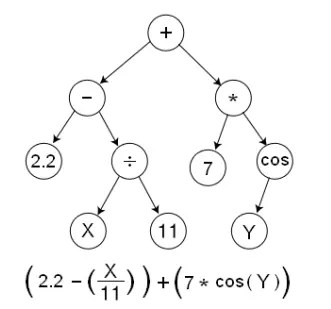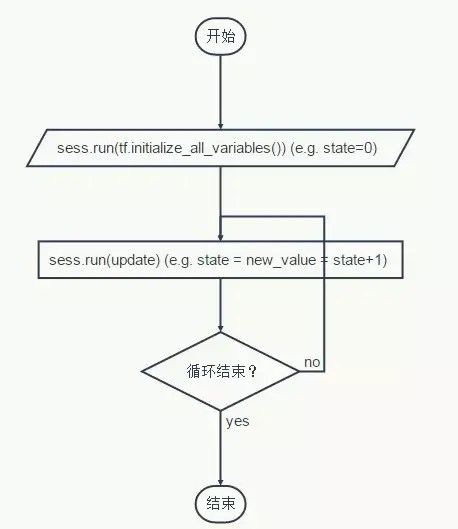# TensorFlow 入门

### CS224d－Day 2:

Day 2 先认识 TensorFlow，了解一下基本用法，下一次就写代码来训练模型算法，以问题为导向，以项目为驱动。

• 1. TensorFlow 是什么
• 2. 为什么需要 TensorFlow
• 3. TensorFlow 的优点
• 4. TensorFlow 的工作原理
• 5. 安装
• 6. TensorFlow 基本用法
• 要点
• 例子
• 概念
• 张量
• 会话

### 1. TensorFlow 是什么

Tensor(张量)意味着 N 维数组，Flow(流)意味着基于数据流图的计算，TensorFlow即为张量从图的一端流动到另一端。

### 4. TensorFlow 的工作原理

TensorFlow是用数据流图(data flow graphs)技术来进行数值计算的。### 5. 安装

Anaconda 是一个集成许多第三方科学计算库的 Python 科学计算环境，用 conda 作为自己的包管理工具，同时具有自己的计算环境，类似 Virtualenv。

• 安装 Anaconda
我之前已经安装过 Anaconda 了，直接从下面进行：
• 建立一个 conda 计算环境
``````# 计算环境名字叫 tensorflow:
# Python 2.7
\$ conda create -n tensorflow python=2.7``````
• 激活环境，使用 conda 安装 TensorFlow
``````\$ source activate tensorflow
(tensorflow)\$  # Your prompt should change

# Mac OS X, CPU only:
• 安装成功后，每次使用 TensorFlow 的时候需要激活 conda 环境
• conda 环境激活后，你可以测试是否成功，在终端进入 python，输入下面代码，没有提示错误，说明安装 TensorFlow 成功：
``````\$ python
...
>>> import tensorflow as tf
>>> hello = tf.constant('Hello, TensorFlow!')
>>> sess = tf.Session()
>>> print(sess.run(hello))
Hello, TensorFlow!
>>> a = tf.constant(10)
>>> b = tf.constant(32)
>>> print(sess.run(a + b))
42
>>>``````
• 当你不用 TensorFlow 的时候，关闭环境:
``````(tensorflow)\$ source deactivate

\$  # Your prompt should change back``````
• 再次使用的时候再激活:
``````\$ source activate tensorflow
(tensorflow)\$  # Run Python programs that use TensorFlow.
...

(tensorflow)\$ source deactivate``````

### 6. TensorFlow 基本用法

• 将计算流程表示成图；
• 通过Sessions来执行图计算；
• 将数据表示为tensors；
• 使用Variables来保持状态信息；
• 分别使用feeds和fetches来填充数据和抓取任意的操作结果；

``````# (tensorflow)\$ python   用 Python API 写 TensorFlow 示例代码

import tensorflow as tf
import numpy as np

# 用 NumPy 随机生成 100 个数据
x_data = np.float32(np.random.rand(2, 100))
y_data = np.dot([0.100, 0.200], x_data) + 0.300

# 构造一个线性模型
b = tf.Variable(tf.zeros())
W = tf.Variable(tf.random_uniform([1, 2], -1.0, 1.0))
y = tf.matmul(W, x_data) + b

# 最小化方差
loss = tf.reduce_mean(tf.square(y - y_data))
train = optimizer.minimize(loss)

# 初始化变量
init = tf.initialize_all_variables()

# 启动图 (graph)
sess = tf.Session()
sess.run(init)

# 拟合平面
for step in xrange(0, 201):
sess.run(train)
if step % 20 == 0:
print step, sess.run(W), sess.run(b)

# 输出结果为：
0 [[-0.14751725  0.75113136]] [ 0.2857058]
20 [[ 0.06342752  0.32736415]] [ 0.24482927]
40 [[ 0.10146417  0.23744738]] [ 0.27712563]
60 [[ 0.10354312  0.21220125]] [ 0.290878]
80 [[ 0.10193551  0.20427427]] [ 0.2964265]
100 [[ 0.10085492  0.201565  ]] [ 0.298612]
120 [[ 0.10035028  0.20058727]] [ 0.29946309]
140 [[ 0.10013894  0.20022322]] [ 0.29979277]
160 [[ 0.1000543   0.20008542]] [ 0.29992008]
180 [[ 0.10002106  0.20003279]] [ 0.29996923]
200 [[ 0.10000814  0.20001261]] [ 0.29998815]``````

``````W = tf.Variable(tf.random_uniform([1, 2], -1.0, 1.0))

y = tf.matmul(W, x_data) + b

init = tf.initialize_all_variables()

sess = tf.Session()
sess.run(init)

sess.run(train)
print step, sess.run(W), sess.run(b)``````

• TensorFlow 用图来表示计算任务，图中的节点被称之为operation，缩写成op。
• 一个节点获得 0 个或者多个张量 tensor，执行计算，产生0个或多个张量。
• 图必须在会话(Session)里被启动，会话(Session)将图的op分发到CPU或GPU之类的设备上，同时提供执行op的方法，这些方法执行后，将产生的张量(tensor)返回。

1. 构建图

``````import tensorflow as tf

# 创建一个 常量 op, 返回值 'matrix1' 代表这个 1x2 矩阵.
matrix1 = tf.constant([[3., 3.]])

# 创建另外一个 常量 op, 返回值 'matrix2' 代表这个 2x1 矩阵.
matrix2 = tf.constant([[2.],[2.]])

# 创建一个矩阵乘法 matmul op , 把 'matrix1' 和 'matrix2' 作为输入.
# 返回值 'product' 代表矩阵乘法的结果.
product = tf.matmul(matrix1, matrix2)``````

2. 张量 Tensor

3. 在一个会话中启动图

``````# 启动默认图.
sess = tf.Session()

# 调用 sess 的 'run()' 方法, 传入 'product' 作为该方法的参数，
# 触发了图中三个 op (两个常量 op 和一个矩阵乘法 op)，
# 向方法表明, 我们希望取回矩阵乘法 op 的输出.
result = sess.run(product)

# 返回值 'result' 是一个 numpy `ndarray` 对象.
print result
# ==> [[ 12.]]

# 任务完成, 需要关闭会话以释放资源。
sess.close()``````

``````# 进入一个交互式 TensorFlow 会话.
import tensorflow as tf
sess = tf.InteractiveSession()

x = tf.Variable([1.0, 2.0])
a = tf.constant([3.0, 3.0])

# 使用初始化器 initializer op 的 run() 方法初始化 'x'
x.initializer.run()

# 增加一个减法 sub op, 从 'x' 减去 'a'. 运行减法 op, 输出结果
sub = tf.sub(x, a)
print sub.eval()
# ==> [-2. -1.]``````

Tensorflow 的变量必须先初始化，然后才有值！而常值张量是不需要的。

``````# －创建一个变量, 初始化为标量 0.  初始化定义初值
state = tf.Variable(0, name="counter")

# 创建一个 op, 其作用是使 state 增加 1
one = tf.constant(1)
update = tf.assign(state, new_value)

# 启动图后, 变量必须先经过`初始化` (init) op 初始化,
# 才真正通过Tensorflow的initialize_all_variables对这些变量赋初值
init_op = tf.initialize_all_variables()

# 启动默认图, 运行 op
with tf.Session() as sess:

# 运行 'init' op
sess.run(init_op)

# 打印 'state' 的初始值
# 取回操作的输出内容, 可以在使用 Session 对象的 run() 调用 执行图时,
# 传入一些 tensor, 这些 tensor 会帮助你取回结果.
# 此处只取回了单个节点 state，
# 也可以在运行一次 op 时一起取回多个 tensor:
# result = sess.run([mul, intermed])
print sess.run(state)

# 运行 op, 更新 'state', 并打印 'state'
for _ in range(3):
sess.run(update)
print sess.run(state)

# 输出:

# 0
# 1
# 2
# 3``````Ok，总结一下，来一个清晰的代码：

``````import tensorflow as tf

# 建图
matrix1 = tf.constant([[3., 3.]])
matrix2 = tf.constant([[2.],[2.]])

product = tf.matmul(matrix1, matrix2)

# 启动图
sess = tf.Session()

# 取值
result = sess.run(product)
print result

sess.close()``````

TensorFlow和普通的Numpy的对比
cs224d的课件中有下面这个代码，来看一下二者之间的区别：eval()

``````In : a = np.zeros((2,2))

In : print(a)
[[ 0.  0.]
[ 0.  0.]]``````

``````In : ta = tf.zeros((2,2))

In : print(ta)
Tensor("zeros_1:0", shape=(2, 2), dtype=float32)

In : print(ta.eval())
[[ 0.  0.]
[ 0. 0.]]``````

Day 1 宏观了解了 NLP，Day 2 搞定了工具，下次要直接先进入实战，训练模型，先从 Logistic 和 NN 开始，一边看模型一边写代码一边思考模型原理，这样理解才会更深刻！